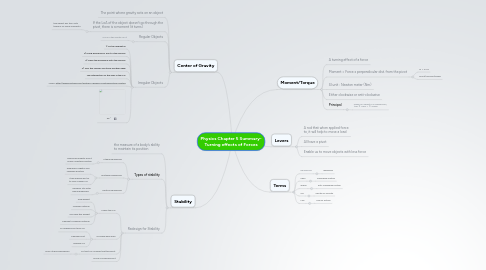# Physics Chapter 5 Summary- Turning effects of Forces

Get Started. It's FreePhysics Chapter 5 Summary- Turning effects of Forces## 1. Moment/Torque

### 1.2. Moment = Force x perpendicular dist. from the pivot

1.2.1. M = F x d

1.2.2. Pivot/Fulcrum/Hinge

### 1.5. Principal

1.5.1. When an object is in equilibrium, the ∑ cwm = ∑ acwm

## 2. Terms

2.1.1. Balanced

### 2.2. cwm

2.2.1. clockwise motion

### 2.3. acwm

2.3.1. anti-clockwise motion

### 2.4. CG

2.4.1. Center of Gravity

### 2.5. LoA

2.5.1. Line of Action

## 4. Center of Gravity

### 4.2. If the LoA of the object doesn't go through the pivot, there is a moment (it turns)

4.2.1. the object will turn until there is no more moments

### 4.3. Regular Objects

4.3.1. CG is in the center on it

### 4.4. Irregular Objects

4.4.1. 1) Set up apparatus

4.4.2. 2) Hang plumbline in front of the laminar

4.4.3. 3) Trace the plumbline onto the laminar

4.4.4. 4) Turn the laminar and trace another edge

4.4.5. The intersection of the lines is the CG

4.4.7. Eg ^

## 5. Stability

### 5.2. Types of stability

5.2.1. Stable Equilibrium

5.2.1.1. Displaced Slightly and it is able maintain position

5.2.2. Unstable Equilibrium

5.2.2.1. Displaced Slightly and changes position

5.2.2.2. It will always will try to find a lower CG

5.2.3. Neutral Equilibrium

5.2.3.1. Remains still after being displaced

### 5.3. Redesign for Stability

5.3.1. Lower the CG

5.3.1.2. Change material

5.3.1.3. Increase the weight

5.3.1.4. Change to heavier material

5.3.2. Increase base area

5.3.2.1. To change pivot and CG

5.3.2.2. Change pivot

5.3.2.3. change CG

5.3.3. So that CG is lower that the pivot

5.3.3.1. More Stable equilibrium

5.3.4. Move CG below pivot